### Home > MC2 > Chapter 2 > Lesson 2.3.3 > Problem2-118

2-118.

Copy and complete each of the Diamond Problems below. The pattern used in the Diamond Problems is shown below.1.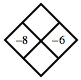What is $(−8)(−6)$?
What is $(−8)+(−6)$?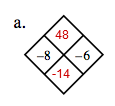1.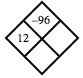$(12) (?) = −96$ value times $12$ will result in $−96$?

What is $12+(−8)$?

1.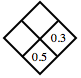See (b).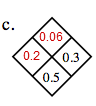1.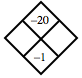What $2$ numbers add to $−1$ and multiply to get $−20$?